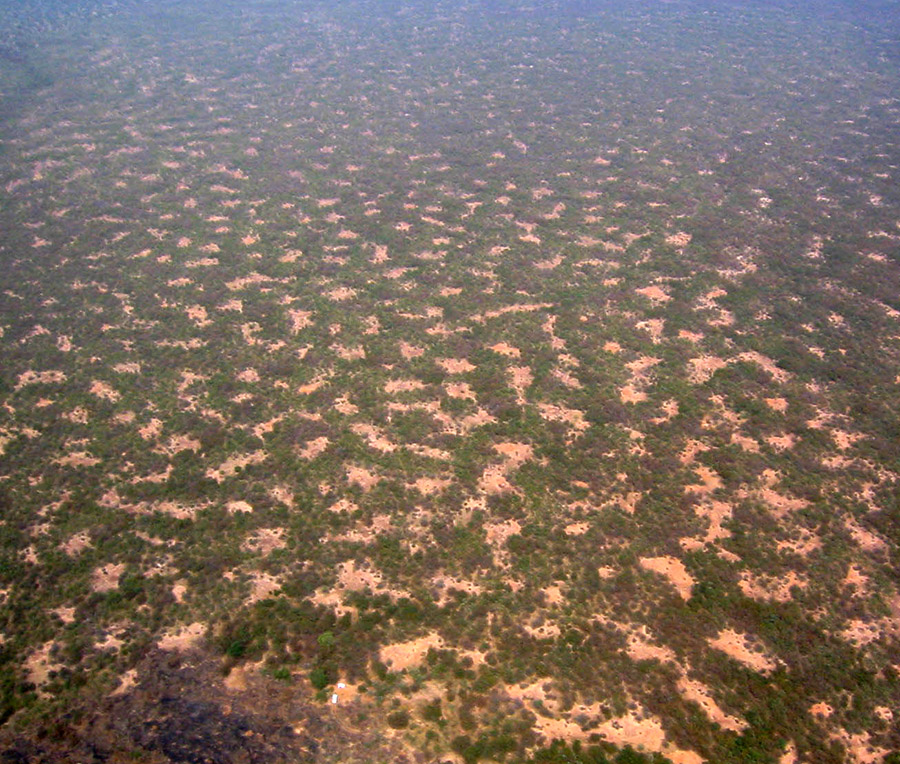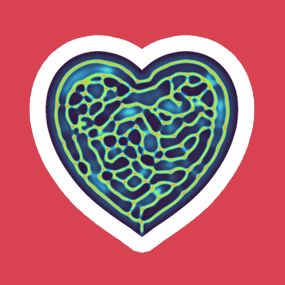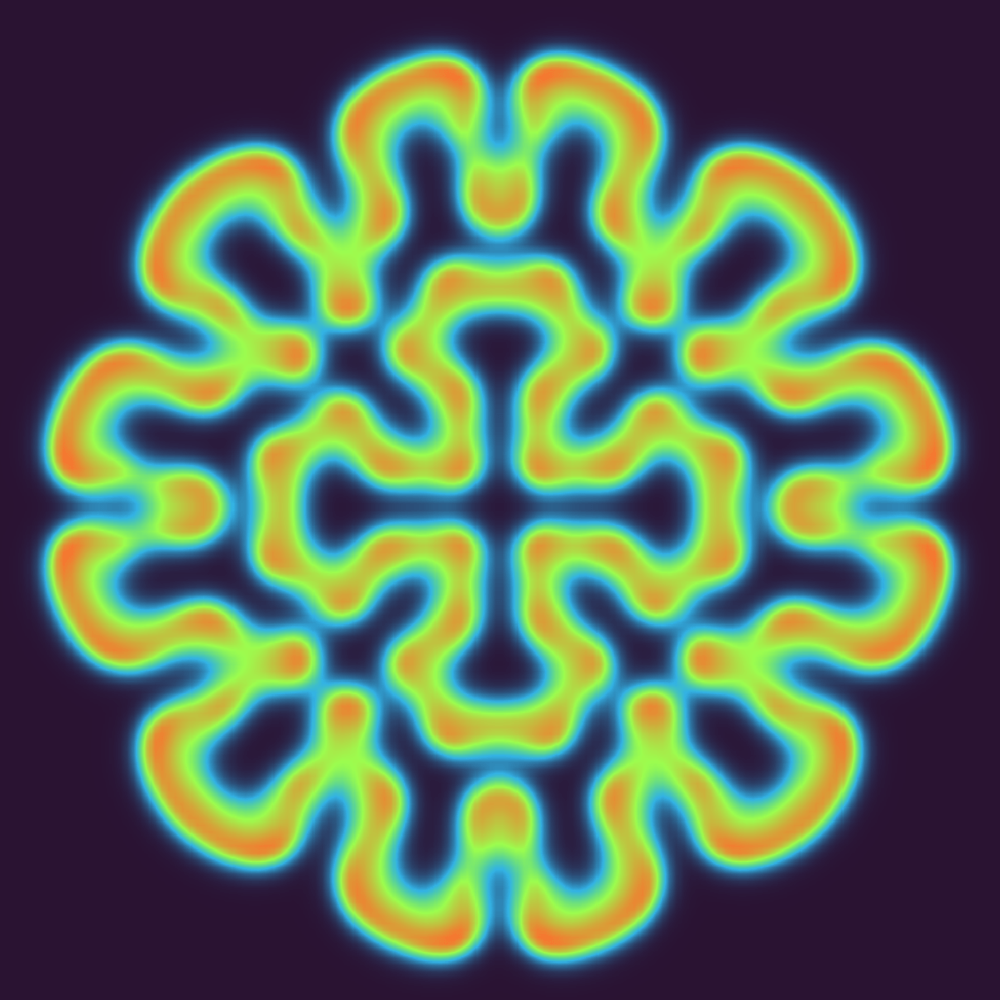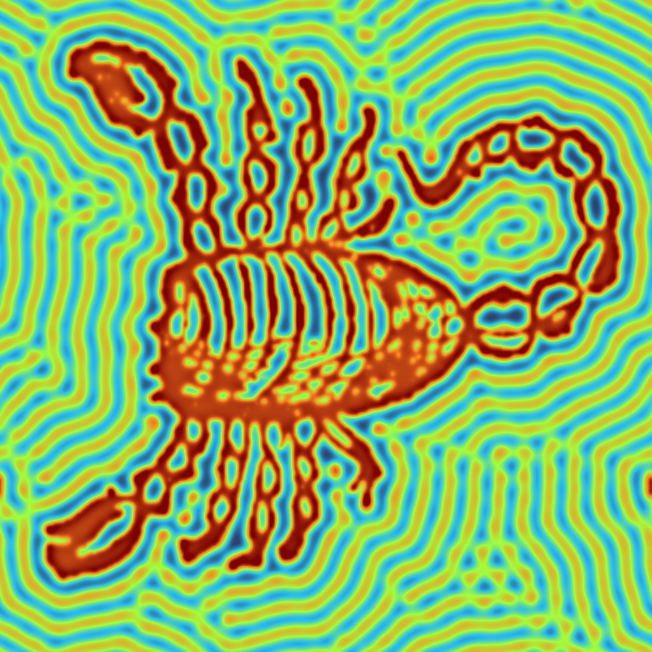# On the cover: VisualPDE.com

Ben Walker, Adam Townsend & Andrew Krause invite you to play with their fun online PDE visualiserOur whole world is constantly changing, and this can be modelled by differential equations: equations that link together quantities with the rate at which they are changing. If you’re in a hot room and your friend opens the window in the middle of winter, the room will cool down. The colder it is outside, the faster the room cools down.

Mathematically we could say the change in temperature in the room ($T$) over time ($t$) is proportional to the difference between the indoor and outdoor temperature,
$\frac{\mathrm{d}T}{\mathrm{d} t} = \alpha (T-T_{\text{outside}}).$
That’s a differential equation in time. But this isn’t the full story! If you stand closer to the window, you’re going to feel colder: heat spreads in space as well as in time.

Differential equations that combine changes over time and space (or just multiple variables in general) are known as partial differential equations, or PDEs. It just so happens that one of the coolest (or hottest?) PDEs is the one that describes how heat spreads out in time and space ($x$):
$\frac{\partial T}{\partial t} = D \frac{\partial^2 T}{\partial x^2}.$
The interesting thing is that this model also works for anything that likes to spread out, not just heat, and in as many dimensions as you want. Bacteria on surfaces, chemicals in water, people on the Metro… all spreading out (technically assuming some sort of randomness) can be captured in this, the diffusion equation.

But if we’re describing bacteria spreading out, in addition to diffusion, we might also want to include reproduction. How quickly do bacteria reproduce? A simple model is growth proportional to the current population size, $u(x,t)$! That gives us, for some parameters $D$ and $\alpha$,
$\frac{\partial u}{\partial t} = D\frac{\partial^2 u}{\partial x^2} + \alpha u.$
But why stop there? We could have different sorts of reproduction rules, and even extra species! How about some predator–prey dynamics of rabbits, $u$, being chased by foxes, $v$, in two-dimensional space?
\begin{align*}
\frac{\partial u}{\partial t} &= D_u \nabla^2 u+au-buv,\\
\frac{\partial v}{\partial t} &= D_v \nabla^2 v+cuv-dv.
\end{align*}
These sorts of systems—‘diffusion + something’ —are called reaction–diffusion systems, and they really are everywhere in mathematical modelling of physics, biology and chemistry.

Not only that, the solutions are totally not boring. They can pulsate, move around, and collide in complicated ways! But for us to appreciate this, we need to be able to see it.

And that is actually quite a challenge. Because the solutions are functions of space (often 2D) and time, they are hard to draw on paper. But nature has already solved this problem! If we are modelling two competing populations of plants in a desert (say a green plant and a white plant), we could climb a big rock, look down from above and see where each plant is growing. Darker spots means more of the green plant, and lighter spots means more white plant. All we have to do to see the solution is then wait. And wait. And wait. And so long as we’re not eaten by a snake, we’ll see the whole solution in time.Aerial view of a Gapped bush plateau in the W National Park, Niger. Image: Nicolas Barbier, CC BY-SA 3.0.

It is reasonable not to want to risk death by desert snake, so instead we can just plot a solution in 2D by getting a computer to colour in a rectangle and then change the colouring over a few seconds…

…so long as we can get the computer to (a) plot, (b) make videos, and (c) actually solve the system! Because the other downside is that solutions to these systems are hard, and sometimes impossible, to find. We can do a pretty good job with numerical approximations using a computer, though, but even approximations can be awkward to set up and require a lot of tuning on top of technical programming knowledge. And what if all you want to do is understand what changing a single parameter does?

This is where our new website, VisualPDE.com, comes in. We made it for our own interest and we think you might like it too. Think Desmos for PDEs, except it makes fun pictures that the cover art for this issue is based on. And it does so instantaneously, right before your eyes!

Here’s a solution to the diffusion equation:We started with heat in the centre of the square and then let the heat diffuse outwards. We’ve chosen to make red hot and blue cold, but you can pick the colour scheme that works best for you. But the other images are more exciting—what about them? Read on!

You might have heard about recent trends in scientific computing where we give graphics cards work to do, instead of the standard processing chip (the CPU). Your phone or your computer displays boomer memes or fascinating Chalkdust articles on the screen by telling each pixel on the screen what colour to be. It’s a big grid. And we can numerically approximate a PDE solution as well by treating the space we’re solving on—for example, our desert floor—as a big, but really fine, grid. On this grid, the derivatives become differences (it’s replacing $\mathrm{d} y/\mathrm{d} x$ by $\mathrm{\Delta} y/\mathrm{\Delta} x$) and the maths becomes simple.

The nice new stuff is that before, you’d have to get your CPU to solve the (even simple) maths on its own grid, and then display it on the screen. This is slow. Today, you can get the graphics card to do the maths itself, directly displaying the solution on the screen. It’s lightning fast!

This is what VisualPDE.com does. We use Javascript, a WebGL library and a low-level language called GLSL so that you can write your equations in your browser, on your phone or computer, and then see the solution evolve in time. It works instantly. You can even prod it with your finger to change the current solution.

OK, but maybe you think we are interested in this just because it’s our research (it is) or because we teach this stuff to undergraduates (we do). But really it’s because the solutions are really pretty! Just look at these!You might not know that the first run at looking at these solutions was done by codebreaker Alan Turing—yes, this is Alan Turing’s Other Work! Let’s take a look at some fun examples, which we invite you to play with on VisualPDE.com.

## Artificial life

The Gray–Scott system, given by
\begin{align*}
\frac{\partial u}{\partial t}&=\nabla^2 u+u^2v – (a+b)u,\\
\frac{\partial v}{\partial t}&=D\nabla^2v-u^2v + a(1-v),
\end{align*}
has been heavily studied as a model of pattern formation, and even as a model of ‘artificial life’, analogous to Conway’s game of life. Many websites and research papers classify the different things it can do. As the values $a$ and $b$ vary, this system can exhibit waves, oscillations, stationary and moving spots, holes, or chaotic oscillations. Here’s one behaviour when $D=2$:As with non-artificial life, the model gets weirder and more fascinating the more you study it!

## Beating hearts

The cover is inspired by the FitzHugh–Nagumo model, pictured at the front of this article,
\begin{align*}
\frac{\partial u}{\partial t}&=\nabla^2 u +u-u^3-v,\\
\frac{\partial v}{\partial t}&=D\nabla^2v+ \varepsilon_v(u-a_v v-a_z).
\end{align*}
Originally, it was a simple model of voltage in the squid giant axon (not to be confused with the giant squid axon). It has since been used to model electrical activity in the heart, as well as formation of patterns in contexts including the folds and wrinkles of the brain. The model can do several things such as: relax to a uniform state, oscillate uniformly, form fixed patterns, and form moving patterns (typically spiral waves and/or chaos). In the example you can find on VisualPDE.com, we’ve set the model to allow both uniform oscillations and stationary patterns, so you get a competition between the two, with one behaviour winning if you tweak the parameters slightly. This scenario is not just mathematically interesting: scar tissue in heart muscles has been shown to prevent waves of excitation from moving through the heart. These patterns are pretty but deadly!

## Your face as a PDE

Models can contain functions of just space, $S(x,y)$. Maybe $S$ is the location of nutrient-rich soil for plants or dangerous terrain for animals. But why not let $S$ represent a picture? And why not let that picture be your face? We adapt the so-called Schnakenberg model,
\begin{align*}
\frac{\partial u}{\partial t} &= D_u \nabla^2 u + (1-S)-u+u^2v,\\
\frac{\partial v}{\partial t} &= D_v \nabla^2 v + 1-u^2 v,
\end{align*}
where the brighter your picture is, the higher the value of $S$. Behold the glorious scorpion picture!Visit VisualPDE.com on your phone, take a selfie, and see your face as a PDE pattern!

## What can you find?

So we invite you to have a play! Head over to VisualPDE.com and see what cool pictures and effects you get when you adjust the parameters on different models. If you’re techy, it’s all open source so you can see how we did it on GitHub. We’d love to see what you can do!Ben is a lecturer & 1851 research fellow at the University of Bath. He has enjoyed this project taking over his life.Adam is an assistant professor at Durham University, where he investigates weird, non-Newtonian fluids. If he’s not talking about the maths of chocolate fountains he is probably thinking about fonts, helping Professor Dirichlet answer your personal problems, and/or listening to BBC Radio 2.Andrew is an assistant professor in the department of mathematical sciences at Durham University. He has enjoyed coming up with increasingly horrible systems to simulate on VisualPDE.